$$\newcommand{\id}{\mathrm{id}}$$ $$\newcommand{\Span}{\mathrm{span}}$$ $$\newcommand{\kernel}{\mathrm{null}\,}$$ $$\newcommand{\range}{\mathrm{range}\,}$$ $$\newcommand{\RealPart}{\mathrm{Re}}$$ $$\newcommand{\ImaginaryPart}{\mathrm{Im}}$$ $$\newcommand{\Argument}{\mathrm{Arg}}$$ $$\newcommand{\norm}{\| #1 \|}$$ $$\newcommand{\inner}{\langle #1, #2 \rangle}$$ $$\newcommand{\Span}{\mathrm{span}}$$

# 2.E: Review Exercises and Sample Exam

$$\newcommand{\vecs}{\overset { \rightharpoonup} {\mathbf{#1}} }$$ $$\newcommand{\vecd}{\overset{-\!-\!\rightharpoonup}{\vphantom{a}\smash {#1}}}$$$$\newcommand{\id}{\mathrm{id}}$$ $$\newcommand{\Span}{\mathrm{span}}$$ $$\newcommand{\kernel}{\mathrm{null}\,}$$ $$\newcommand{\range}{\mathrm{range}\,}$$ $$\newcommand{\RealPart}{\mathrm{Re}}$$ $$\newcommand{\ImaginaryPart}{\mathrm{Im}}$$ $$\newcommand{\Argument}{\mathrm{Arg}}$$ $$\newcommand{\norm}{\| #1 \|}$$ $$\newcommand{\inner}{\langle #1, #2 \rangle}$$ $$\newcommand{\Span}{\mathrm{span}}$$ $$\newcommand{\id}{\mathrm{id}}$$ $$\newcommand{\Span}{\mathrm{span}}$$ $$\newcommand{\kernel}{\mathrm{null}\,}$$ $$\newcommand{\range}{\mathrm{range}\,}$$ $$\newcommand{\RealPart}{\mathrm{Re}}$$ $$\newcommand{\ImaginaryPart}{\mathrm{Im}}$$ $$\newcommand{\Argument}{\mathrm{Arg}}$$ $$\newcommand{\norm}{\| #1 \|}$$ $$\newcommand{\inner}{\langle #1, #2 \rangle}$$ $$\newcommand{\Span}{\mathrm{span}}$$

## Review Exercises

Exercise $$\PageIndex{1}$$ Introduction to Algebra

Evaluate.

1. $$2x+7$$, where $$x=−4$$
2. $$−4x+1$$, where $$x=−2$$
3. $$\frac{2}{3}y−\frac{1}{2}$$, where $$y=\frac{3}{5}$$
4. $$−\frac{3}{4}y+\frac{5}{3}$$, where $$y=\frac{2}{3}$$
5. $$b^{2}−4ac$$, where $$a=5, b=−2$$, and $$c=\frac{1}{2}$$
6. $$b^{2}−4ac$$, where $$a=−\frac{1}{4}, b=−1$$, and $$c=−3$$
7. $$2x^{2}−x+3$$, where $$x=−3$$
8. $$5x^{2}−2x+4$$, where $$x=−1$$
9. Calculate the simple interest earned on a $$3$$-year investment of $$$750$$ at an annual interest rate of $$8$$%. 10. A bus traveled for $$1\frac{2}{3}$$ hours at an average speed of $$48$$ miles per hour. What distance did the bus travel? 11. Calculate the area of a rectangle with dimensions $$4\frac{1}{2}$$ feet by $$6$$ feet. 12. Calculate the volume of a rectangular box with dimensions $$4\frac{1}{2}$$ feet by $$6$$ feet by $$1$$ foot. Answer 1. $$−1$$ 3. $$−\frac{1}{10}$$ 5. $$−6$$ 7. $$24$$ 9.$$$180$$

11. $$27$$ square feet

Exercise $$\PageIndex{2}$$ Simplifying Algebraic Expressions

Multiply.

1. $$−5(3x−2)$$
2. $$(6x−9)⋅3$$
3. $$\frac{3}{4}(4x^{2}−8x+32)$$
4. $$−20(\frac{1}{10}x^{2}−\frac{2}{5}x−\frac{5}{4})$$
5. $$−(3a−2b+5c−1)$$
6. $$−6(y^{3}+3y^{2}−7y+5)$$

1. $$−15x+10$$

3. $$3x^{2}−6x+24$$

5. $$−3a+2b−5c+1$$

Exercise $$\PageIndex{3}$$ Simplifying Algebraic Expressions

Simplify.

1. $$5a−7b−3a+5b$$
2. $$6x^{2}−4x+7x^{2}−3x$$
3. $$\frac{3}{5}xy+\frac{1}{2}−\frac{1}{10}xy−\frac{1}{4}$$
4. $$−\frac{3}{4}a−\frac{4}{21}b+\frac{1}{3}a−\frac{1}{7}b$$
5. $$a^{2}b+2ab^{2}−7a^{2}b+9ab^{2}$$
6. $$y^{2}−3y+5−y^{2}+9$$
7. $$−8(8x−3)−7$$
8. $$7−(6x−9)$$
9. $$2(3x^{2}−2x+1)−(5x−7)$$
10. $$(2y^{2}+6y−8)−(5y^{2}−12y+1)$$
11. $$6−3(a−2b)+7(5a−3b)$$
12. $$10−5(x^{2}−x+1)−(3x^{2}+5x−1)$$
13. Subtract $$5x−1$$ from $$2x−3$$.
14. Subtract $$x−3$$ from twice the quantity $$x−1$$.

1. $$2a−2b$$

3. $$\frac{1}{2}xy+\frac{1}{4}$$

5. $$−6a^{2}b+11ab^{2}$$

7. $$−64x+17$$

9. $$6x^{2}−9x+9$$

11. $$32a−15b+6$$

13. $$−3x−2$$

Exercise $$\PageIndex{4}$$ Solving Linear Equations: Part 1

Is the given value a solution to the linear equation?

1. $$−x+3=−18; x=−15$$
2. $$4x−3=−3x; x=−2$$
3. $$8x+2=5x+1; x=−\frac{1}{3}$$
4. $$2x+4=3x−2; x=−1$$

1. No

3. Yes

Exercise $$\PageIndex{5}$$ Solving Linear Equations: Part 1

Solve.

1. $$y+23=25$$
2. $$−3x=54$$
3. $$\frac{x}{4}=8$$
4. $$\frac{5}{2}x=\frac{2}{3}$$
5. $$7x−5=−54$$
6. $$−2x+7=43$$
7. $$7x+3=0$$
8. $$4x+5=5$$
9. $$1=10−3x$$
10. $$10−5y=15$$
11. $$7−y=28$$
12. $$33−x=16$$
13. $$\frac{5}{6}x+\frac{1}{3}=\frac{3}{2}$$
14. $$−\frac{2}{3}y+\frac{1}{5}=−\frac{1}{3}$$
15. The sum of $$9x$$ and $$6$$ is $$51$$.
16. The difference of $$3x$$ and $$8$$ is $$25$$.

1. $$2$$

3. $$32$$

5. $$−7$$

7. $$−\frac{3}{7}$$

9. $$3$$

11. $$−21$$

13. $$\frac{7}{5}$$

15. $$5$$

Exercise $$\PageIndex{6}$$ Solving Linear Equations: Part II

Solve.

1. $$5x−2=3x+6$$
2. $$7x+1=2x−29$$
3. $$14x+1=15x−11$$
4. $$6y−13=3+7y$$
5. $$8y+6−3y=22−3y$$
6. $$12−5y+6=y−6$$
7. $$5−2(7x−1)=2x+1$$
8. $$10−5(x−1)=5−x$$
9. $$2x−(3x−4)=7−x$$
10. $$9x−3(2x+1)=3x−3$$
11. $$2(5x−2)−3(2x+1)=5(x−3)$$
12. $$3(5x−1)−4(x−4)=−5(2x+10)$$
13. $$\frac{3}{2}(4x−3)+\frac{1}{4}=1$$
14. $$\frac{3}{4}−\frac{1}{6}(4x−9)=2$$
15. $$\frac{2}{3}(9x−3)+\frac{1}{2}=3(2x−\frac{1}{2})$$
16. $$1−\frac{5}{4}(4x−1)=5(\frac{1}{2}−x)$$
17. The sum of $$4x$$ and $$3$$ is equal to the difference of $$7x$$ and $$8$$.
18. The difference of $$5x$$ and $$1$$ is equal to the sum of $$12x$$ and $$1$$.
19. Solve for $$x$$: $$y=9x+1$$
20. Solve for $$y$$: $$5x+2y=3$$
21. Solve for $$l$$: $$P=2l+2w$$
22. Solve for $$b$$: $$A=\frac{1}{2}bh$$

1. $$4$$

3. $$12$$

5. $$2$$

7. $$\frac{3}{8}$$

9. $$Ø$$

11. $$8$$

13. $$\frac{7}{8}$$

15. $$R$$

17. $$\frac{11}{3}$$

19. $$x=\frac{y-1}{9}$$

21. $$l=\frac{P−2w}{2}$$

Exercise $$\PageIndex{7}$$ Applications of Linear Equations

1. A larger integer is $$3$$ more than twice a smaller integer. If their sum is $$39$$, then find the integers.
2. A larger integer is $$5$$ more than $$3$$ times a smaller integer. If their sum is $$49$$, then find the integers.
3. The sum of three consecutive odd integers is $$45$$. Find the integers.
4. The sum of three consecutive even integers is $$72$$. Find the integers.
5. The sum of three consecutive integers is $$60$$. Find the integers.
6. The length of a rectangle is $$7$$ centimeters less than twice its width. If the perimeter measures $$46$$ centimeters, then find the dimensions of the rectangle.
7. A triangle has sides whose measures are consecutive even integers. If the perimeter is $$24$$ meters, then find the measure of each side.
8. The circumference of a circle measures $$24π$$ inches. Find the radius of the circle.
9. Mary invested $$$1,800$$ in two different accounts. One account earned $$3.5$$% simple interest and the other earned $$4.8$$%. If the total interest after $$1$$ year was$$$79.25$$, then how much did she invest in each account?
10. James has $$$6$$ in dimes and quarters. If he has $$4$$ fewer quarters than he does dimes, then how many of each coin does he have? 11. Two brothers leave the house at the same time traveling in opposite directions. One averages $$40$$ miles per hour and the other $$36$$ miles per hour. How long does it take for the distance between them to reach $$114$$ miles? 12. Driving to her grandmother’s house, Jill made several stops and was only able to average $$40$$ miles per hour. The return trip took $$2$$ hours less time because she drove nonstop and was able to average $$60$$ miles per hour. How long did it take Jill to drive home from her grandmother’s house? Answer 1. $$12, 27$$ 3. $$13, 15, 17$$ 5. $$19, 20, 21$$ 7. $$6$$ meters, $$8$$ meters, $$10$$ meters 9. Mary invested$$$550$$ at $$3.5$$% and $$$1,250$$ at $$4.8$$%. 11. They will be $$114$$ miles apart in $$1\frac{1}{2}$$ hours. Exercise $$\PageIndex{8}$$ Ratio and Proportion Applications Solve. 1. $$\frac{3}{4}=\frac{n}{8}$$ 2. $$\frac{7}{3}=\frac{2}{8n}$$ 3. $$\frac{6}{n}=\frac{30}{11}$$ 4. $$\frac{n}{5}=\frac{2}{3}$$ 5. $$\frac{3n−1}{3}=\frac{1}{2}$$ 6. $$\frac{4}{2n+5}=−\frac{1}{3}$$ 7. $$−3=\frac{1}{n−1}$$ 8. $$\frac{2}{n−6}=\frac{1}{2n+1}$$ 9. Find two numbers in the proportion $$4$$ to $$5$$ whose sum is $$27$$. 10. A larger number is $$2$$ less than twice a smaller number. If the two numbers are in the proportion $$5$$ to $$9$$, then find the numbers. 11. A recipe calls for $$1\frac{1}{2}$$ teaspoons of vanilla extract for every $$3$$ cups of batter. How many teaspoons of vanilla extract should be used with $$7$$ cups of batter? 12. The ratio of female to male employees at a certain bank is $$4$$ to $$5$$. If there are $$80$$ female employees at the bank, then determine the total number of employees. Answer 1. $$6$$ 3. $$\frac{11}{5}$$ 5. $$\frac{5}{6}$$ 7. $$\frac{2}{3}$$ 9. $$12, 15$$ 11. $$3\frac{1}{2}$$ teaspoons Exercise $$\PageIndex{9}$$ Ratio and Proportion Applications If triangle $$ABC$$ is similar to triangle $$RST$$, then find the remaining two sides given the following. 1. $$a=4, b=9, c=12$$, and $$s=3$$ 2. $$b=7, c=10, t=15$$, and $$r=6$$ 3. At the same time of day, a pole casts a $$27$$-foot shadow and $$4$$-foot boy casts a $$6$$-foot shadow. Calculate the height of the pole. 4. An equilateral triangle with sides measuring $$10$$ units is similar to another equilateral triangle with scale factor of $$2:3$$. Find the perimeter of the unknown triangle. Answer 1. $$t = 4, r = \frac{4}{3}$$ 3. $$18$$ feet Exercise $$\PageIndex{10}$$ Introduction to Inequalities and Interval Notation Graph all solutions on a number line and provide the corresponding interval notation. 1. $$x<-1$$ 2. $$x\leq 10$$ 3. $$x\geq 0$$ 4. $$x>-2$$ 5. $$-\frac{1}{2}\leq x<\frac{3}{2}$$ 6. $$-20<x<30$$ 7. $$x<5\text{ or }x\geq 15$$ 8. $$x<2\text{ or }x>0$$ Answer 1. $$(−∞, −1)$$Figure 2.E.1 3. $$[0, ∞)$$Figure 2.E.2 5. $$[−\frac{1}{2}, \frac{3}{2})$$Figure 2.E.3 7. $$(−∞, 5)∪[15, ∞)$$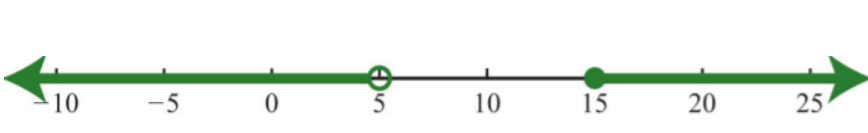Figure 2.E.4 Exercise $$\PageIndex{11}$$ Introduction to Inequalities and Interval Notation Determine the inequality given the answers expressed in interval notation. 1. $$(−∞, 3)$$ 2. $$[−4, ∞)$$ 3. $$(−2, 2)$$ 4. $$(−3, 8]$$ 5. $$(−∞, 1)∪[3, ∞)$$ 6. $$(−∞, −8]∪[8, ∞)$$ Answer 1. $$x<3$$ 3. $$−2<x<2$$ 5. $$x<1\text{ or }x\geq 3$$ Exercise $$\PageIndex{12}$$ Linear Inequalities (One Variable) Solve and graph. In addition, present the solution set in interval notation. 1. $$x+2>−1$$ 2. $$−4x≥16$$ 3. $$9x+4≤-5$$ 4. $$5x−7<13$$ 5. $$7x+5-8x\geq 15$$ 6. $$5x-6+3x<2+9x-5$$ 7. $$3x-(x-4)>x+4$$ 8. $$3(2x−1)−3(x−2)≤2(x+4)$$ 9. $$2−5(x−4)>12$$ 10. $$3x−5(x−2)≥11−5x$$ 11. $$−1<2x+5≤11$$ 12. $$−2≤\frac{1}{4}x−\frac{7}{2}≤2$$ 13. $$5x+3<−2\text{ or }6x−5≥7$$ 14. $$20−3x≤5\text{ or }5−2x≥25$$ Answer 1. $$x>−3; (−3, ∞)$$Figure 2.E.5 3. $$x≤−1; (−∞, −1]$$Figure 2.E.6 5. $$x≤−10; (−∞, −10]$$Figure 2.E.7 7. $$x>0; (0, ∞)$$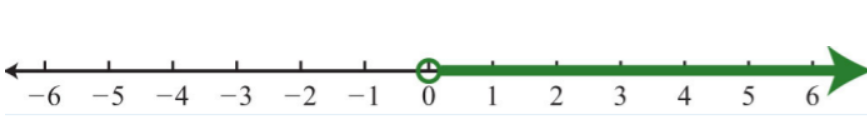Figure 2.E.8 9. $$x<2; (−∞, 2)$$Figure 2.E.9 11. $$−3<x\leq 3; (-3,3]$$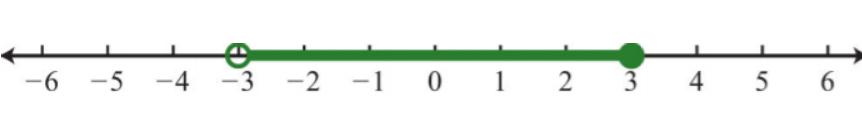Figure 2.E.10 13. $$x<−1 \text{ or }x≥2; (−∞, −1)∪[2, ∞)$$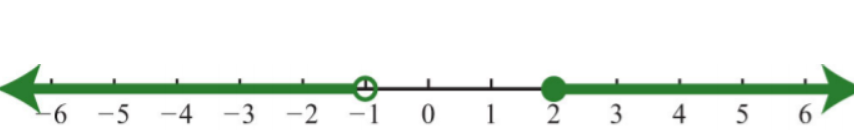Figure 2.E.11 ## Sample Exam Exercise $$\PageIndex{13}$$ 1. Evaluate $$b^{2}−4ac$$, where $$a=−1, b=−2\, and \(c=\frac{1}{2}$$. 2. Determine the area of a triangle given that the base measures $$10$$ centimeters and the height measures $$5$$ centimeters. $$(A=\frac{1}{2}bh)$$ Answer 1. $$6$$ Exercise $$\PageIndex{14}$$ Simplify. 1. $$5−2(4x−1)$$ 2. $$\frac{1}{4}x−\frac{2}{3}y+\frac{1}{2}x−\frac{3}{5}y$$ 3. $$(5a+4ab−2b)−(3a+2ab−3b)$$ 4. $$3x−(x^{2}+5x−1)+(x^{2}−x+4)$$ Answer 1. $$−8x+7$$ 3. $$2ab+2a+b$$ Exercise $$\PageIndex{15}$$ Solve. 1. $$2−5x=27$$ 2. $$\frac{1}{2}x−\frac{3}{4}=−\frac{1}{8}$$ 3. $$5x−7=3x−5$$ 4. $$3(y−3)−(4y+2)=1$$ 5. $$5(x−2)−3(x+2)=2x−3$$ 6. $$\frac{5}{8}=\frac{n}{32}$$ 7. $$\frac{3}{n+1}=−\frac{6}{4}$$ 8. Solve for $$b$$: $$A=a+2b$$. Answer 1. $$−5$$ 3. $$1$$ 5. $$Ø$$ 7. $$−3$$ Exercise $$\PageIndex{16}$$ Solve and graph the solution set. In addition, present the solution set in interval notation. 1. $$2x+3>23$$ 2. $$5(−2x+1)≤35$$ 3. $$4(3x−2)<3(2x+1)+1$$ 4. $$−9≤3(x+4)≤21$$ 5. $$6(x−\frac{1}{3})<−2\text{ or }\frac{1}{5}(x+10)≥3$$ Answer 1. $$x>10; (10, ∞)$$Figure 2.E.12 3. $$x<2; (−∞, 2)$$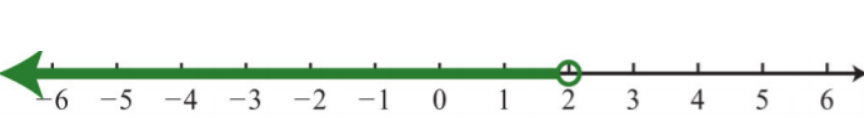Figure 2.E.13 5. $$x<0\text{ or } x≥5; (−∞, 0)∪[5, ∞)$$\)Figure 2.E.14 Exercise $$\PageIndex{17}$$ 1. An algebra student earns $$75, 79$$, and $$89$$ points on the first three quizzes. What must she score on the fourth quiz to earn an average of at least $$80$$? 2. The sum of three consecutive odd integers is $$117$$. Find the integers. 3. The length of a rectangle is $$6$$ inches less than twice the width. If the perimeter measures $$39$$ inches, then find the dimensions of the rectangle. 4. Millie invested her$$$5,350$$ savings in two accounts. One account earns $$5$$% annual interest and the other earns $$6.2$$% in annual interest. If she earned $$$317.30$$ simple interest in $$1$$ year, then how much was in each account? 5. Because of traffic, Joe was only able to drive an average of $$42$$ miles per hour on the trip to a conference. He was able to average $$63$$ miles per hour on the return trip and it took $$1$$ hour less time. How long did it take Joe to drive home from the conference? 6. A graphic designer wishes to crop an image in the width-to-height ratio of $$3:2$$. If the height is required to be $$400$$ pixels, then to how many pixels should the width be set? Answer 2. The three odd integers are $$37, 39$$, and $$41$$. 4. Millie invested$$$1,200$$ in the account earning $$5$$% annual interest and \$$$4,150$$ in the account earning $$6.2$$%.

6. The width should be set to $$600$$ pixels.